# Test: Number Systems, Boolean Algebra & Sequential Logic Circuits

## 20 Questions MCQ Test GATE ECE (Electronics) 2023 Mock Test Series | Test: Number Systems, Boolean Algebra & Sequential Logic Circuits

Description
Attempt Test: Number Systems, Boolean Algebra & Sequential Logic Circuits | 20 questions in 60 minutes | Mock test for Railways preparation | Free important questions MCQ to study GATE ECE (Electronics) 2023 Mock Test Series for Railways Exam | Download free PDF with solutions
QUESTION: 1

Solution:
QUESTION: 2

### If (211)x = (152)8 , then the value of base x is

Solution:

In general, since we are comfortable dealing with Decimal system, the best way to go about under the circumstances is to convert one Number into decimal Number and then with the base x, convert another Number into Decimal Number and equate them to find the value of x.

So, (152)8 is 64*1+8*5+2, which is 106 in Decimal System.

(211)x is 2x^2+ 1*x+1 in Decimal System.

Now, we solve 2x^2+x+1 = 106

i. e. 2x^2+x-105=0

Therefore 2x^2–14x+15x-105=0

Therefore 2x(x-7)+15(x-7)=0

Therefore (x-7)(2x+15)=0

Therefore either x = 7 or x = -15/2.

Obviously x = -15/2 isnot the answer. So, x = 7 is the answer, which can be very easily verified. 2*7^2+1*7+1 = 106 which issame as (152)8.

Thus the base for 211 is 7.

QUESTION: 3

### 11001, 1001 and 111001 correspond to the 2’s complement representation of the following set of numbers

Solution:

All are 2’s complement of 7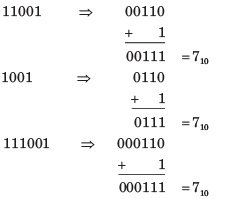QUESTION: 4

A signed integer has been stored in a byte using 2’s complement format. We wish to store the same integer in 16-bit word. We should copy the original byte to the less significant byte of the word and fill the more significant byte with

Solution:

See a example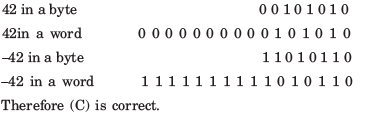QUESTION: 5

A computer has the following negative numbers stored in binary form as shown. The wrongly stored number is

Solution:
QUESTION: 6

The circuit shown in fig. is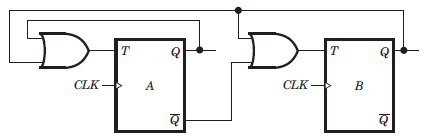Solution: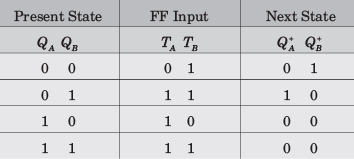From table it is clear that it is a MOD–3 counter.

QUESTION: 7

The counter shown in fig. is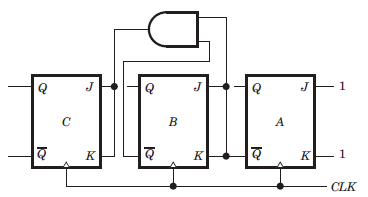Solution:

It is a down counter because 0 state of previous FFs change the state of next FF. You may trace the following sequence, let initial state be000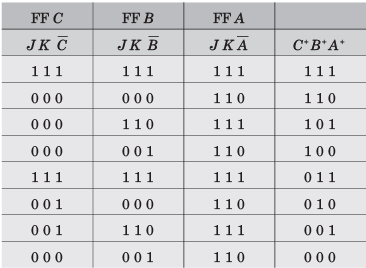QUESTION: 8

The counter shown in fig. counts from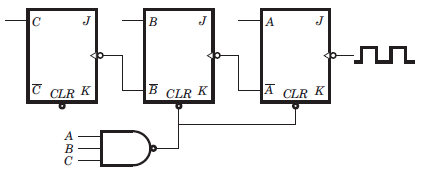Solution:

It is a down counter because the inverted FF output drive the clock inputs. The NAND gate will clear FFs A and B when the count tries to recycle to 111. This will produce as result of 100. Thus the counting sequence will be 100, 011, 010, 001, 000, 100 etc.

QUESTION: 9

The mod-number of the asynchronous counter shown in fig. is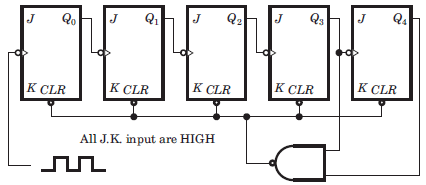Solution:

It is a 5 bit ripple counter. At 11000 the output of NAND gate is LOW. This will clear all FF. So it is a Mod 24 counter. Note that when 11000 occur, the CLR input is activated and all FF are immediately cleared. So it is a MOD 24 counter not MOD 25.

QUESTION: 10

The frequency of the pulse at z in the network shown in fig. is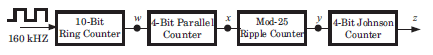Solution:

10-bit ring counter is a MOD–10, so it divides the 160 kHz input by 10. therefore, w = 16 kHz. The four-bit parallel counter is a MOD–16. Thus, the frequency at x = 1 kHz. The MOD–25 ripple counter produces a frequency at y = 40 Hz. (1 kHz/25 = 40 Hz).
The four-bit Johnson Counter is a MOD-8. This, the frequency at z = 5 Hz.

QUESTION: 11

The three-stage Johnson counter as shown in fig. is clocked at a constant frequency of fc from the starting state of Q2 Q1Q0 = 101. The frequency of output Q2 Q1Q0 will be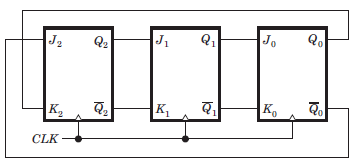Solution: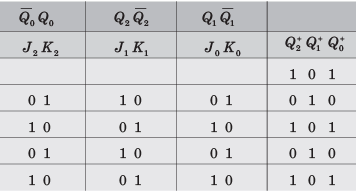We see that 1 0 1 repeat after every two cycles, hence frequency will be fc/2

QUESTION: 12

The counter shown in the fig. has initially Q2Q1Q0 = 000. The status of Q2 Q1Q after the first pulse is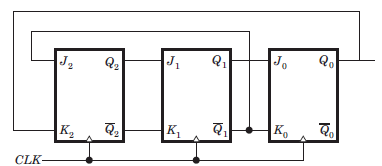Solution:

At first cycle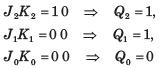QUESTION: 13

A 4 bit ripple counter and a 4 bit synchronous counter are made by flips flops having a propagation delay of 10 ns each. If the worst case delay in the ripple counter and the synchronous counter be R and S respectively, then

Solution:

In ripple counter delay 4Td = 40 ns.

The synchronous counter are clocked simultaneously, then its worst delay will be equal to 10 ns.

QUESTION: 14

A 4 bit modulo–6 ripple counter uses JK flip-flop. If the propagation delay of each FF is 50 ns, the maximum clock frequency that can be used is equal to

Solution:

4 bit uses 4 FF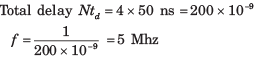QUESTION: 15

The initial contents of the 4-bit serial-in-parallel-out right-shift, register shown in fig. is 0 1 1 0. After three clock pulses are applied, the contents of the shift register will be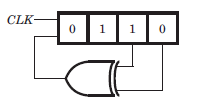Solution: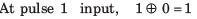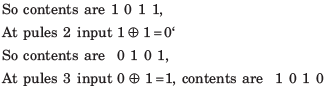QUESTION: 16

Consider the signed binary number A = 01010110 and B = 1110 1100 where B is the 1’s complement and MSB is the sign bit. In list-I operation is given, and in list-II resultant binary number is given.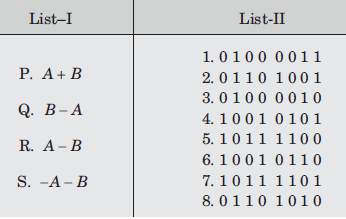The correct match is

Solution: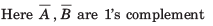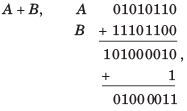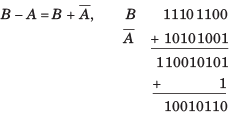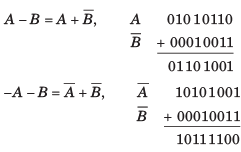QUESTION: 17

The simplified form of a logic function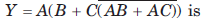Solution: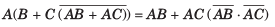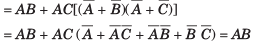QUESTION: 18

If the decimal number is a fraction then its binary equivalent is obtained by ________ the number continuously by 2.

Solution:

On multiplying the decimal number continuously by 2, the binary equivalent is obtained.

QUESTION: 19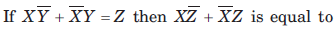Solution: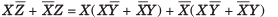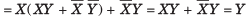QUESTION: 20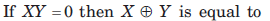Solution: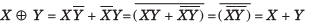Use Code STAYHOME200 and get INR 200 additional OFF Use Coupon Code